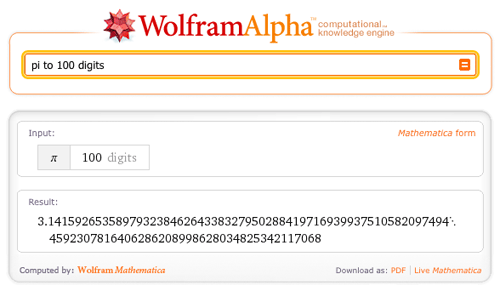August 3, 2011–

In my last blog post on plotting functionality in Wolfram|Alpha, we looked at 2D and 3D Cartesian plotting. In this post, we will look at 2D polar and parametric plotting.

For those of you unfamiliar with polar plots, a point on a plane in polar coordinates is located by determining an angle θ and a radius r. For example, the Cartesian point (x, y) = (1, 1) has the polar coordinates (r, θ) = (√2,π/4). The following diagram illustrates the relationship between Cartesian and polar plots.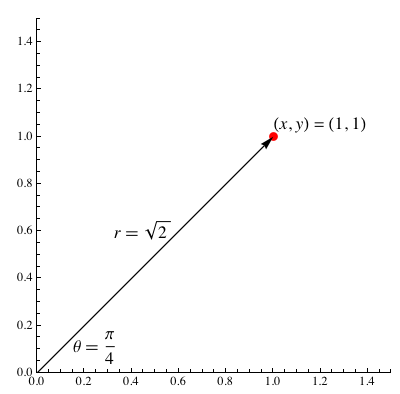To generate a polar plot, we need to specify a function that, given an angle θ, returns a radius r that is a function r(θ). Making a polar plot in Wolfram|Alpha is very easy; for example, we can plot Archimedes’ spiral. More »

July 27, 2011–

In recent dinner conversation with my brother, I commented that I was “99.9 repeating” percent sure that my favorite author, Jorge Luis Borges, had lived into the 1980s (Wolfram|Alpha later showed me that he did, in fact, live through 1986!). I felt confident about my knowledge, but wanted to leave myself a little wiggle room, just in case. My brother grinned at me and said, “I know you know your geometric sequences. If you say you’re 99.9 repeating percent sure, then you’re 100 percent sure.”

I blushed, embarrassed. He was definitely right!

But just what did my brother mean? Well, he reminded me that:

99.999… = 99 + 9/10 + 9/100 + 9/1000 + 9/10000…

Glancing at the above, the series 9/10 + 9/100 + 9/1000 + 9/10000… stands out. Each term in this series is 1/10 times the term before it, making it a geometric series. A geometric series is a series wherein each term in the sequence is a constant number, r, multiplied by the term before it. Any geometric series whose r satisfies -1 < r < 1 is a convergent series, and we can say to what the series converges:

Sum[r^k, {k, 0, ∞}] = 1/(1-r)

March 31, 2011–

Today we are releasing Wolfram Multivariable Calculus and Wolfram Astronomy, the next two apps on a growing list of Wolfram Course Assistant Apps. These course assistants will help students learn their course material using the power of Wolfram|Alpha.

The Wolfram Astronomy Course Assistant allows you to easily look up information on constellations and planets, but it can also calculate anything from the next lunar eclipse to the solar interior.

March 3, 2011–

Students of the history of science will recall learning that Galileo observed and described the periodic motion of a simple pendulum around 1602. Until being supplanted by other technologies around the first third of the 20th century, this property of pendula has been indispensible in the creation of accurate timekeeping devices.

An idealized pendulum consisting of a weight (often called a “bob”) on the end of a massless cord and suspended from a frictionless pivot is called a simple pendulum or, more explicitly, a simple gravity pendulum. Wolfram|Alpha has known about simple pendula for some time, as you can verify by entering “pendulum”. In fact, doing so brings up not one but two pendulum results:

February 25, 2011–

Why did the mathematician name his dog Cauchy? Because he left a residue at every pole!

But could the mathematician find the poles and their residues for a given function? He certainly could, with the help of Wolfram|Alpha.

We are proud to announce that Wolfram|Alpha has added residues and poles to its ever-expanding library of mathematical data that it can compute! To showcase this behavior, let’s first recall just what a pole is.

In the study of complex analysis, a pole is a singularity of a function where the function behaves like 1/zn at z == 0 .

More technically, a point z_0 is a pole of a function if the Laurent series expansion of the function about the point z_0 has only finitely many terms with a negative degree of zz_0. As an example, let’s look at the Laurent expansion of 1/Sin[z] at z == 2 Pi:

September 30, 2010–

In 1977, famed computer scientist Donald Knuth decided he didn’t like the typesetting of the second edition of The Art of Computer Programming. Rather than unhappily accept the results of photographic typesetting techniques, Knuth invented his own digital typesetting solution, TeX, which would eventually become the standard typesetting system for mathematical and academic content. Wikipedia displays math content using a variant of TeX, and research papers from a large range of fields are very commonly submitted in TeX format.

Our team recently added the ability to understand TeX notation and convert it to the Mathematica form used by the powerful Wolfram|Alpha engine. We’ve received many requests for this functionality from people who use Wolfram|Alpha for advanced math and physics. It’s often easy and natural to write mathematics using TeX, whereas it can otherwise be quite difficult to express clearly in plaintext notation.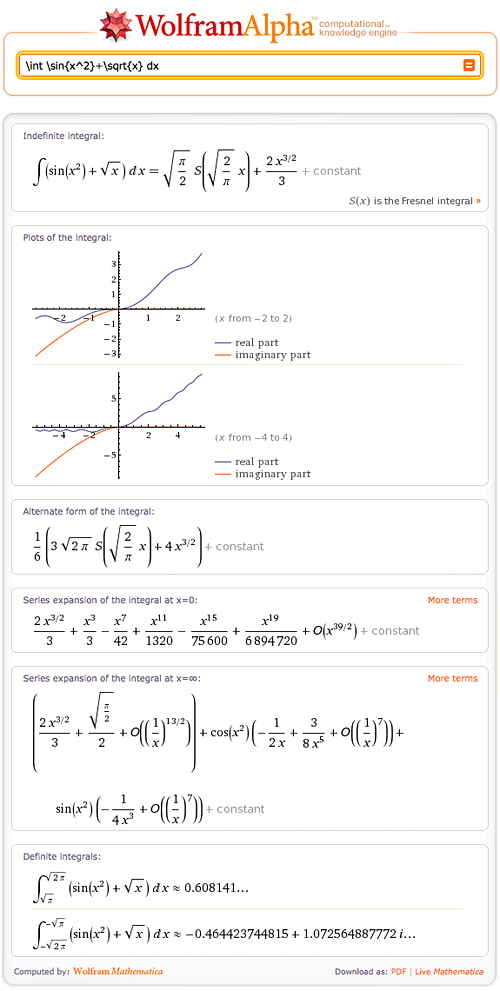The beauty of this new capability is that one can now see, compute, and understand typeset mathematics all through the union of TeX notation and Wolfram|Alpha computation. Complicated expressions are now easily represented using the elegance of TeX: More »

June 15, 2010–

Wolfram|Alpha computes things. While the use of computations to predict the outcomes of scientific experiments, natural processes, and mathematical operations is by no means new (it has become a ubiquitous tool over the last few hundred years), the ease of use and accessibility of a large, powerful, and ever-expanding collection of such computations provided by Wolfram|Alpha is.

Virtually all known processes occur in such a way that certain functionals that describe them become extremal. Typically this happens with the action for time dependent processes and quantities such as the free energy for static configurations. The equations describing the extremality condition of a functional are frequently low-order ordinary and/or partial differential equations and their solutions. For example, for a pendulum: Frechet derivative of Integrate[x'[t]^2/2 – Cos[x[t]], {t, -inf, inf}] wrt x[tau]. Unfortunately, if one uses a sufficiently realistic physical model that incorporates all potentially relevant variables (including things like friction, temperature dependence, deformation, and so forth), the resulting equations typically become complicated—so much so that in most cases, no exact closed-form solution can be found, meaning the equations must be solved using numerical techniques. A simple example is provided by free fall from large heights: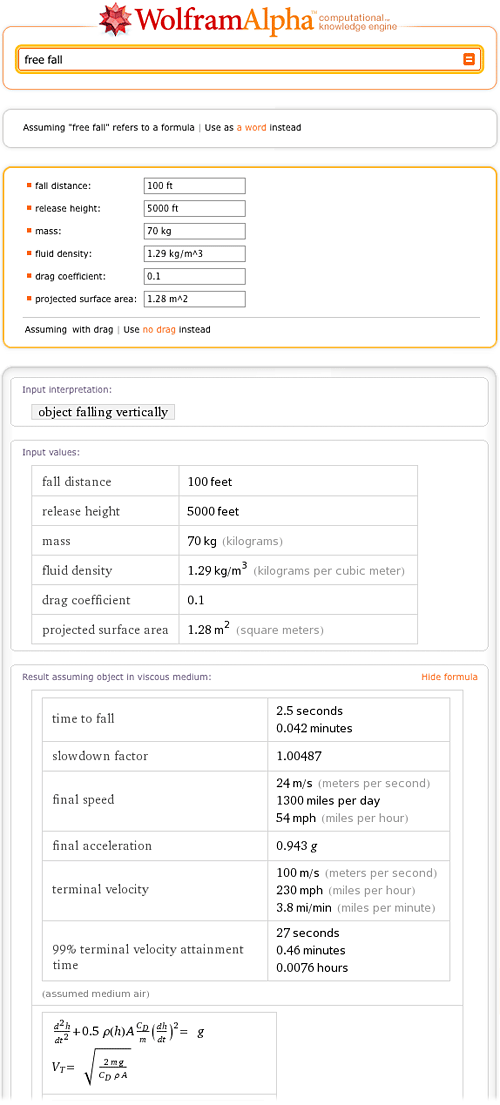On the other hand, some systems, such as the force of a simple spring, can be described by formulas involving simple low-order polynomial or rational relations between the relevant problem variables (in this case, Hooke’s law, F = k x):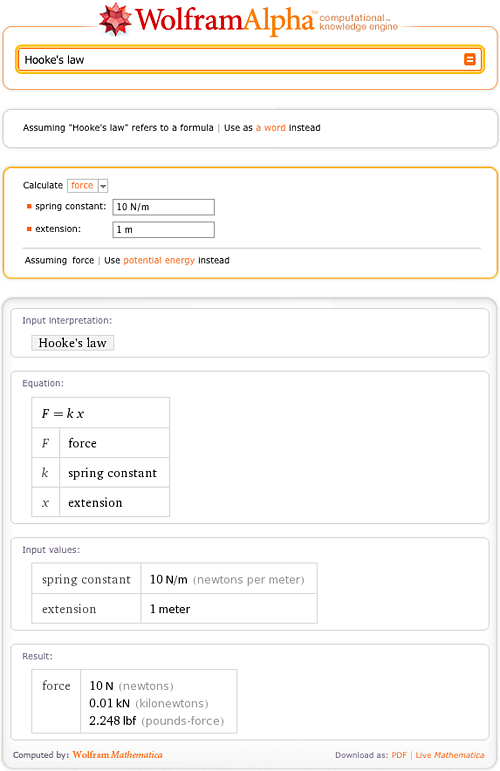Over the last 200+ years, mathematicians and physicists have found a large, fascinating, and insightful world of phenomena that can be described exactly using these so-called special functions (also commonly known as “the special functions of mathematical physics”), the class of functions that describe phenomena between being difficult and complicated. It includes a few hundred members, and can be viewed as an extension of the so-called elementary functions such as exp(z), log(z), the trigonometric functions, their inverses, and related functions.

Special functions turn up in diverse areas ranging from the spherical pendulum in mechanics to inequivalent representations in quantum field theory, and most of them are solutions of first- or second-order ordinary differential equations. Textbooks often contain simple formulas that correspond to a simplified version of a general physical system—sometimes even without explicitly stating the implicit simplifying assumptions! However, it is often possible to give a more precise and correct result in terms of special functions. For instance, many physics textbooks offer a simple formula for the inductance of a circular coil with a small radius: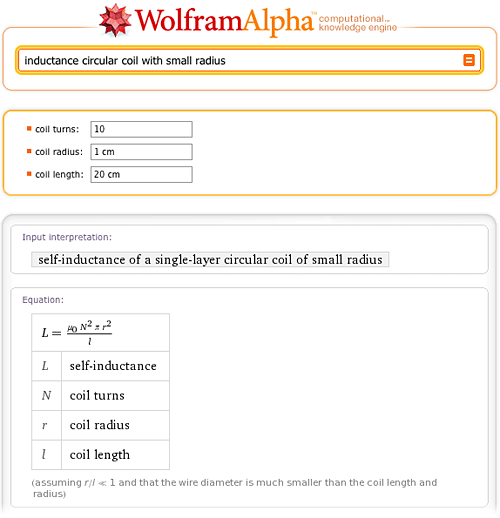While Wolfram|Alpha knows (and allows you to compute with) this simple formula, it also knows the correct general result. In fact, if you just ask Wolfram|Alpha for inductance circular coil, you will be simultaneously presented with two calculators: the one you know from your electromagnetics textbook (small-radius approximation) and the fully correct one. And not only can you compute the results both ways (and see that the results do differ slightly for the chosen parameters, but that the difference can get arbitrarily large), you can also click on the second “Show formula” link (near the bottom of the page on the right side) to see the exact result—which, as can be seen, contains two sorts of special functions, denoted E(m) and K(m) and known as elliptic integrals: More »

May 7, 2010–

Hello, fellow readers of the Wolfram|Alpha Blog—my name’s Justin. In just a few short weeks, I’ll be graduating from the University of Illinois at Urbana-Champaign. Over the years I’ve found my own way of getting things done in regards to homework and studying routines. But this semester I realized there were tools available that would make studying and completing assignments easier and help me understand better. One tool that has become increasingly valuable in my routine and those of other students on my campus is Wolfram|Alpha. Recently, I was invited to share how Wolfram|Alpha is being used by students like myself.

Being a marketing major, I had to take some finance and accounting courses, but I was a bit rusty with the required formulas and the overall understanding of the cash flow concepts, such as future cash flows and the net present values of a future investment. A friend recommended I check out Wolfram|Alpha’s finance tools, and they’ve became indispensable in my group’s casework for the semester. Each proposed future investment we were met with, we would go directly to Wolfram|Alpha to compute the cash flows. We even went as far to show screenshots, such as the one below, of inputs and outputs in our final case presentation last week.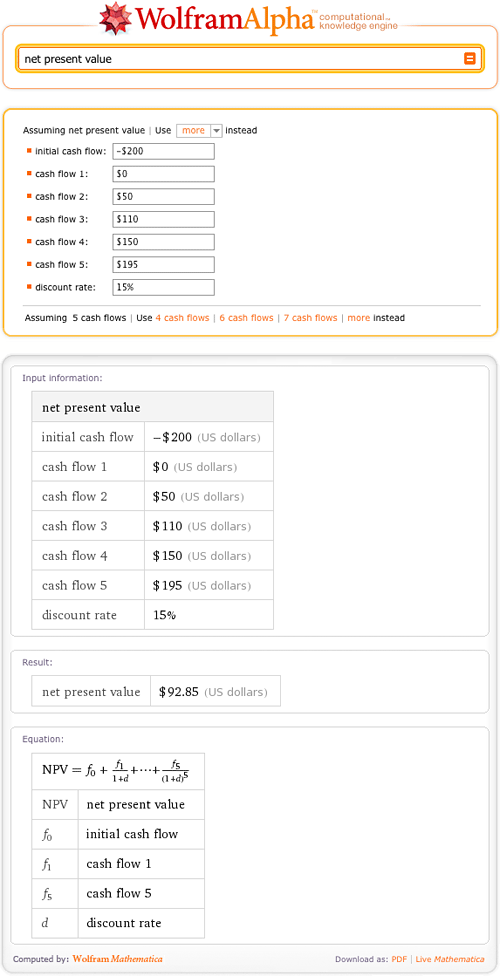I’ve met other students on my campus who have found Wolfram|Alpha to be helpful in their courses. A few months ago while studying in the library, I walked by a table of freshman students all using Wolfram|Alpha on their laptops. I decided to stop and chat with them because I knew one of the girls. They explained how they were using Wolfram|Alpha to model functions and check portions of their math homework. All three girls are enrolled in Calculus III, and not exactly overjoyed about the fact of future— and most likely harder—math classes. More »

March 19, 2010–

Steven Strogatz, a professor of applied mathematics at Cornell University, is currently blogging for The New York Times about issues “from the basics of math to the baffling”. It’s been a fascinating series, starting with preschool math and progressing through subtraction, division, complex numbers, and more. As Wolfram|Alpha is such a powerful tool for working with mathematical concepts, we thought it’d be fun to show how to use it to explore some of the topics in Strogatz’s blog.

First up is Strogatz’s post on “Finding Your Roots”. For a brief introduction to Wolfram|Alpha’s ability to find roots, try “root of 4x+2”.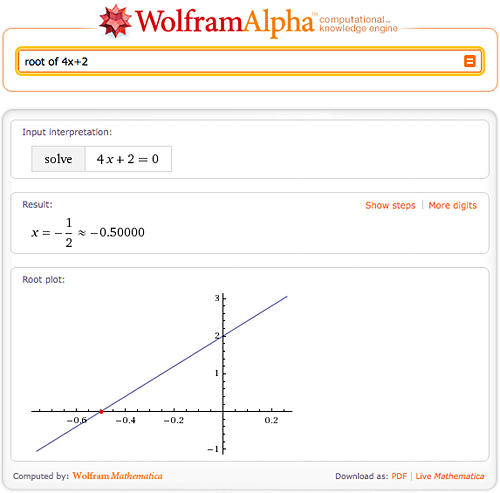Here we found the one and only root of 4x+2, but what if there is more than one root? Not a problem for Wolfram|Alpha—try “4x^2 + 3x – 4”. More »

February 5, 2010–

Version 1.1 of the Wolfram|Alpha App for the iPhone & iPod is now available in the App Store. The new version includes a number of new features that continue to improve the app’s unique mobile Wolfram|Alpha experience. Perhaps its most iconic feature, the specialized keyboards that greet you when you first open the Wolfram|Alpha App, have been painstakingly constructed to ease the burden of entering queries, whether you’re converting from pounds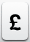to euros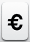or computing a numerical value for the Weierstrass p-function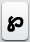. Our goal in creating these keyboards was to form families of characters that naturally occur together both in common use and in traditional mathematical applications. We also wanted mathematical expressions to look and feel natural to enhance usability and understanding. Version 1.1 has four specialized keyboards: the default keyboard, the “math” keyboard accessed by the right-shift key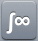, the “Greek” keyboard accessed by one press of the left-shift key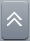, and the “symbol” keyboard accessed by a second press of the left-shift key.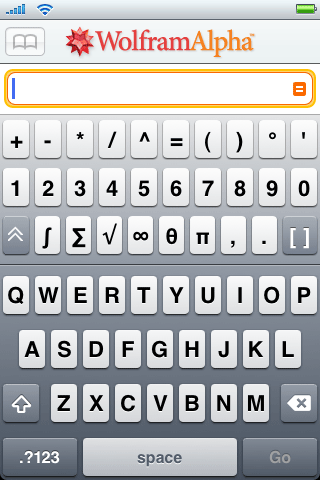To determine the optimal keyboard layout, we scoured Wolfram|Alpha’s server logs for the most commonly entered phrases that have characters with meaning in Wolfram|Alpha. Given that Wolfram|Alpha is built on Mathematica, one of its core strengths is advanced mathematics. True to form most of the commonly typed characters are related to math. For example, you would generally type the word “integrate” to compute an integral on the Wolfram|Alpha website. In the Wolfram|Alpha App you could simply type the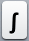key on the math keyboard. The same is true for other symbols common in math, such as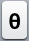and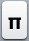. Specifying geometric shapes, such as a triangle, is straightforward as well.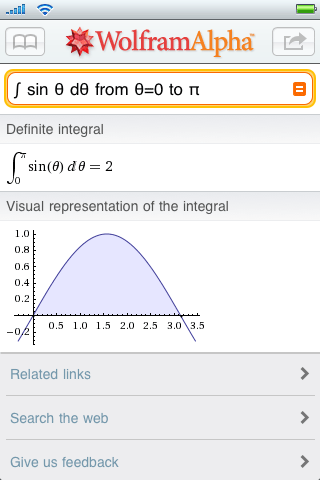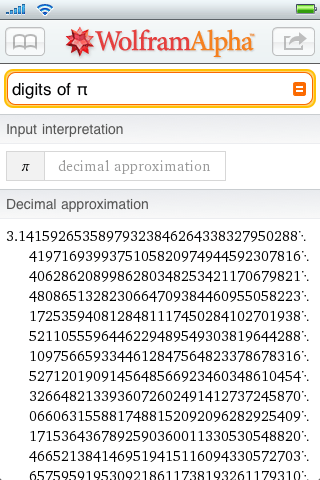More »

December 29, 2009–

Prior to releasing Wolfram|Alpha into the world this past May, we launched the Wolfram|Alpha Blog. Since our welcome message on April 28, we’ve made 133 additional posts covering Wolfram|Alpha news, team member introductions, and “how-to’s” in a wide variety of areas, including finance, nutrition, chemistry, astronomy, math, travel, and even solving crossword puzzles.

As 2009 draws to a close we thought we’d reach into the archives to share with you some of this year’s most popular blog posts.

#### April

Rack ’n’ Roll

Take a peek at our system administration team hard at work on one of the
many pre-launch projects.

#### May

The Secret Behind the Computational Engine in Wolfram|Alpha

Although it’s tempting to think of Wolfram|Alpha as a place to look up facts, that’s only part of the story. The thing that truly sets Wolfram|Alpha apart is that it is able to do sophisticated computations for you, both pure computations involving numbers or formulas you enter, and computations applied automatically to data called up from its repositories.

Why does computation matter? Because computation is what turns generic information into specific answers. Continue reading…

Live, from Champaign!

Wolfram|Alpha just went live for the very first time, running all clusters.

This first run at testing Wolfram|Alpha in the real world is off to an auspicious start, although not surprisingly, we’re still working on some kinks, especially around logging.

While we’re still in the early stages of this long-term project, it is really gratifying to finally have the opportunity to invite you to participate in this project with us. Continue reading…

#### June

Wolfram|Alpha Q&A Webcast

Stephen Wolfram shared the latest news and updates about Wolfram|Alpha and answered several users’ questions in a live webcast yesterday.

If you missed it, you can watch the recording here. Continue reading… More »

December 21, 2009–

We’re really catching the holiday spirit here at Wolfram|Alpha.

We recently announced our special holiday sale for the Wolfram|Alpha app. Now we are launching our first-ever Wolfram|Alpha “Holiday Tweet-a-Day” contest.Here’s how it works.

From tomorrow, Tuesday, December 22, through Saturday, January 2, we’ll use Twitter to give away a gift a day. Be the first to retweet our “Holiday Tweet-a-Day” tweet and you get the prize! You can double your chances to win by following and playing along with Wolfram Research.

Start following us today so you don’t miss your chance to win with our Wolfram|Alpha “Holiday Tweet-a-Day” contest.

December 1, 2009–

## July 17, 2019 Update: Step-by-step solutions has been updated! Learn more.

Have you ever given up working on a math problem because you couldn’t figure out the next step? Wolfram|Alpha can guide you step by step through the process of solving many mathematical problems, from solving a simple quadratic equation to taking the integral of a complex function.

When trying to find the roots of 3x2+x–7=4x, Wolfram|Alpha can break down the steps for you if you click the “Show steps” button in the Result pod.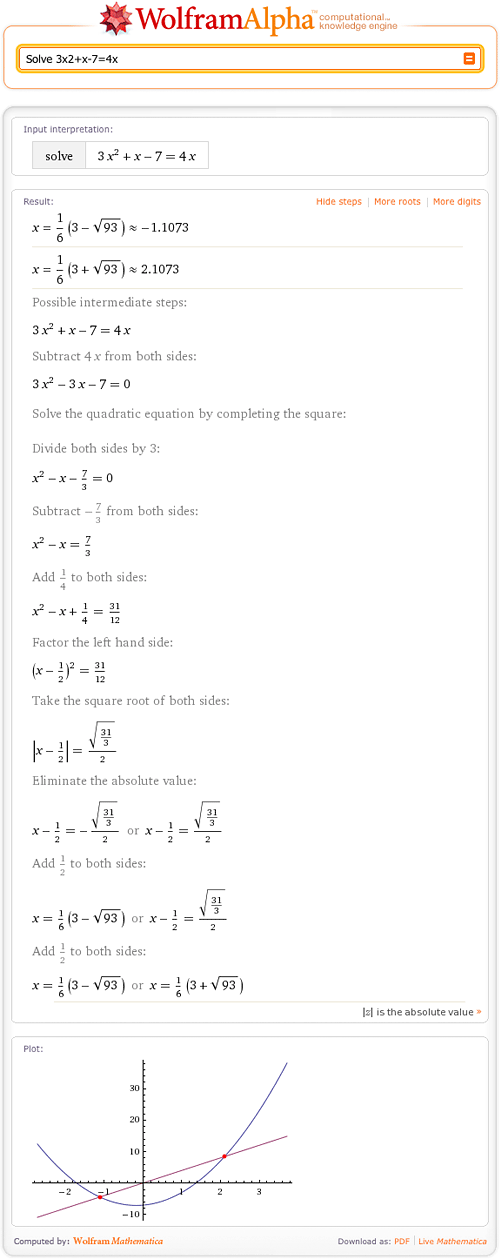As you can see, Wolfram|Alpha can find the roots of quadratic equations. Wolfram|Alpha shows how to solve this equation by completing the square and then solving for x. Of course, there are other ways to solve this problem! More »

September 8, 2009–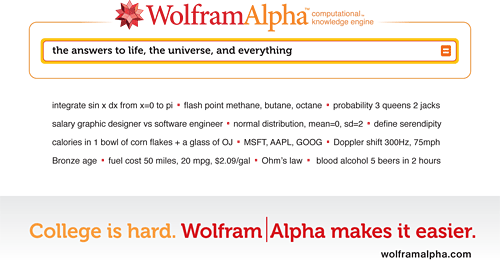We know college is hard. So we’re highlighting examples of how Wolfram|Alpha can make subjects and concepts a bit easier to learn. Wolfram|Alpha is a free computational knowledge engine that can help you tackle everything from calculus, to computing the number of pages for a double-spaced 1000-word essay, to comparing the flash points of methane, butane, and octane, to figuring just how much money it’s going to cost you to drive home to do your laundry. Check out a quick introduction to Wolfram|Alpha from its creator, Stephen Wolfram.

We want to help you take full advantage of this resource. Over the next term, we’ll be highlighting helpful computations and information here on the blog, and even providing ways you can get involved with our company. (Would you like to be a part of the Wolfram|Alpha Team on your campus? Stay tuned to find out how you can be involved.) For this post we selected several of our favorite examples to help you start thinking about how you can use Wolfram|Alpha in your courses, and in your always-changing college life. More »

May 13, 2009–

Some of you have asked whether you’ll be able to use Wolfram|Alpha for challenging math. Of course!